## Final Documentation – HeartBeat/Pulse Sensor – Lie detection maybe?

Description

Create a physically interactive system of your choice that relies on a multimedia computer for some sort of processing or data analysis. The Final should use BOTH Processing AND Arduino. Your focus should be on careful and timely sensing of the relevant actions of the person or people that you’re designing this for, and on clear, prompt, and effective responses. Any interactive system is going to involve systems of listening, thinking, and speaking from both parties. Whether it involves one cycle or many, the exchange should be engaging. You may work alone or in pairs.

Project

I started this project by working with a pulse sensor without thinking about what I would end up with. I progressed by displaying a heartbeat on the screen by using the data I received. I also wanted to work with LEDs, I attached the LEDs with the breadboard. I used two LEDs that turn on and off according to the pulse rate. I also checked the timings between heartbeats by subtracting the last-current, the variable I have used is TBH(time between heartbeats). I also made the heart pump by increasing the stroke weight. You can also use “S” or “s” to save the heartbeat in the folder where the code is saved and later you can compare the heartbeat. To make the pulse rate monitor more interesting, I added some questions to the screen to see how the heartbeat changed with respect to the question, which can be used as a lie detection test. If someone wanted to reset their heartbeats, they can press ‘r’ to reset it, and the monitor displays no heartbeat until some other person uses the sensor. For this project, I used two sensors, out of which one had technical problems. So, I couldn’t use that. But, the idea was that I would compare two people’s heartbeats by asking them questions.

I took some shots during the exhibition of people testing out the monitor and playing the yes/no question game.

The LEDs were covered with a white box that looked like an artificial heart because it would beat every time your pulse was beating. The circuit looked like this: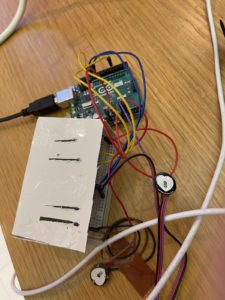Certain challenges:-

1. It took me some time to figure out how the sensor worked because I have never worked with a pulse sensor before.
2. it was hard to display the pulse wave first, then I found some resources to make me understand the concept.
3. it was hard to add further things to the project to make it more useful.

The code is following:-

Arduino

```#define USE_ARDUINO_INTERRUPTS true
#include <PulseSensorPlayground.h>

const int OUTPUT_TYPE = PROCESSING_VISUALIZER;

const int PULSE_SENSOR_COUNT = 2;

const int PULSE_INPUT0 = A0;
const int PULSE_BLINK0 = 13;    //led

const int PULSE_INPUT1 = A1;

const int THRESHOLD = 550;   // to avoid noise when idle

PulseSensorPlayground pulseSensor(PULSE_SENSOR_COUNT);

void setup() {

Serial.begin(250000);

pulseSensor.setSerial(Serial);
pulseSensor.setOutputType(OUTPUT_TYPE);
pulseSensor.setThreshold(THRESHOLD);

if (!pulseSensor.begin()) {

for (;;) {

delay(50);
delay(50);
}
}
}

void loop() {

delay(20);

pulseSensor.outputSample();

for (int i = 0; i < PULSE_SENSOR_COUNT; ++i) {
if (pulseSensor.sawStartOfBeat(i)) {
pulseSensor.outputBeat(i);
}
}
}```

Processing

```import processing.sound.*;
SoundFile file;
import processing.serial.*;
PFont font;

Serial port;
int numSensors = 2; //variable that holds number of sensors
//the varible given below holds certain information
int[] Sensor;
int[] TBH;
int[] BPM;
int[][] RawPPG;
int[][] ScaledPPG;
int[][] ScaledBPM;
float offset;
color bgcolor = color(171,219,227);
int heart[];   //when timing the pulse

//determine the size
int pulseWidth;
int pulseHeight;
int pulseX;
int pulseY[];
int bpmWidth;
int bpmHeight;
int bpmX;
int bpmY[];
int spacer = 10;
boolean beat[]; //boolean to check if heart beat detected

//find serial port
String serialPort;
String[] serialPorts = new String[Serial.list().length];
boolean serialPortFound = false;
int numPorts = serialPorts.length;
boolean refreshPorts = false;
int duration = 1000;
int pressTime;

void setup() {
fullScreen();
frameRate(100);
file = new SoundFile(this, "heart.mp3");
textFont(font);
textAlign(CENTER);
rectMode(CORNER);
ellipseMode(CENTER);
pulseWidth = width-520;
pulseHeight = 1080/numSensors;
pulseX = 10;
pulseY = new int [numSensors];
for(int i=0; i<numSensors; i++){
pulseY[i] = 43 + (pulseHeight * i);
if(i > 0) pulseY[i]+=spacer*i;
}
bpmWidth = 300;
bpmHeight = pulseHeight;
bpmX = pulseX + pulseWidth + 10;
bpmY = new int [numSensors];
for(int i=0; i<numSensors; i++){
bpmY[i] = 43 + (bpmHeight * i);
if(i > 0) bpmY[i]+=spacer*i;
}
heart = new int[numSensors];
beat = new boolean[numSensors];
// Data Variables Setup
Sensor = new int[numSensors];
TBH = new int[numSensors];
BPM = new int[numSensors];
RawPPG = new int[numSensors][pulseWidth];
ScaledPPG = new int[numSensors][pulseWidth];
ScaledBPM = new int [numSensors][bpmWidth];
//setting lines to 0
resetDataTraces();

background(0);
noStroke();
drawDataWindows();
drawHeart();

fill(bgcolor);
listAvailablePorts();
}

void draw() {

if(serialPortFound){
// run only when port connected
background(0);
drawDataWindows();
drawPulseWaveform();
drawBpmWave();
drawHeart();
printDataToScreen();

if(spacePressed){
text("The following game involves Yes/No questions.\n Press 1 to view the first question.", 670, 500);
}
if(onePressed){
text("Are you happy with your life?", 700, 500);
}
if(twoPressed){
text("Have you ever truly been in love?", 700, 500);
}
if(threePressed){
text("Do you think you're successful in life?", 700, 500);
}
if(fourPressed){
text("Have you ever done something unforgivable?", 750, 500);
}
if(fivePressed){
text("Would you save your mother over 17 other random people?", 700, 500);
}
if(sixPressed){
text("Are you a good person?", 700, 500);
}
if(sevenPressed){
text("If you died tomorrow, would you have regrets?", 750, 500);
}

} else {
autoScanPorts(); //scan to find port

if(refreshPorts){
refreshPorts = false;
drawDataWindows();
drawHeart();
listAvailablePorts();
}

for(int i=0; i<numPorts+1; i++){
}

}

}

void drawDataWindows(){
noStroke();
fill(bgcolor);
for(int i=0; i<numSensors; i++){
rect(pulseX, pulseY[i], pulseWidth, pulseHeight);
rect(bpmX, bpmY[i], bpmWidth, bpmHeight);
}
}

void drawPulseWaveform(){
for (int i=0; i<numSensors; i++) {
RawPPG[i][pulseWidth-1] = (1023 - Sensor[i]);

for (int j = 0; j < pulseWidth-1; j++) {
RawPPG[i][j] = RawPPG[i][j+1];
float dummy = RawPPG[i][j] * 0.625/numSensors;
offset = float(pulseY[i]);
ScaledPPG[i][j] = int(dummy) + int(offset);
}
stroke(250, 0, 0);
noFill();
beginShape();
for (int x = 1; x < pulseWidth-1; x++) {
vertex(x+10, ScaledPPG[i][x]);
}
endShape();
}

}

void drawBpmWave(){
//draw bpm wave

for (int i=0; i<numSensors; i++) {
if (beat[i] == true) {
file.play();
beat[i] = false;

for (int j=0; j<bpmWidth-1; j++) {
ScaledBPM[i][j] = ScaledBPM[i][j+1];
}

BPM[i] = constrain(BPM[i], 0, 200);                     // limit the highest BPM value to 200
float dummy = map(BPM[i], 0, 200, bpmY[i]+bpmHeight, bpmY[i]);
ScaledBPM[i][bpmWidth-1] = int(dummy);
}
}
// graph heart rate in small window
stroke(250, 0, 0);                          // color of heart rate graph
strokeWeight(2);                          // thicker line is easier to read
noFill();

for (int i=0; i<numSensors; i++) {
beginShape();
for (int j=0; j < bpmWidth; j++) {
vertex(j+bpmX, ScaledBPM[i][j]);
}
endShape();
}
}
void drawHeart(){
//make the heart and make it beat
fill(250,0,0);
stroke(250,0,0);
int bezierZero = 0;
for(int i=0; i<numSensors; i++){

heart[i]--;
heart[i] = max(heart[i], 0);
if (heart[i] > 0) {
strokeWeight(8);
}
smooth();   //draw hearts
bezier(width-100, bezierZero+70, width-20, bezierZero, width, bezierZero+160, width-100, bezierZero+170);
bezier(width-100, bezierZero+70, width-190, bezierZero, width-200, bezierZero+160, width-100, bezierZero+170);
strokeWeight(1);          // reset the strokeWeight for next time
bezierZero += bpmHeight+spacer;
}
}

void listAvailablePorts(){
//println(Serial.list());
serialPorts = Serial.list();
fill(0);
textFont(font,16);
textAlign(LEFT);

int yPos = 0;

for(int i=numPorts-1; i>=0; i--){
text(serialPorts[i],50, 100+(yPos*20));
yPos++;
}
int p = numPorts;
fill(233,0,0);
text("Refresh Serial Ports List",50, 100+(yPos*20));

textFont(font);
textAlign(CENTER);
}

void autoScanPorts(){
if(Serial.list().length != numPorts){
if(Serial.list().length > numPorts){
println("New Ports Opened!");
int diff = Serial.list().length - numPorts;
serialPorts = expand(serialPorts,diff);
numPorts = Serial.list().length;
}else if(Serial.list().length < numPorts){
println("Some Ports Closed!");
numPorts = Serial.list().length;
}
refreshPorts = true;
return;
}
}

void resetDataTraces(){
for (int i=0; i<numSensors; i++) {
BPM[i] = 0;
for(int j=0; j<bpmWidth; j++){
ScaledBPM[i][j] = bpmY[i] + bpmHeight;
}
}
for (int i=0; i<numSensors; i++) {
Sensor[i] = 512;
for (int j=0; j<pulseWidth; j++) {
RawPPG[i][j] = 1024 - Sensor[i];
}
}
}

void printDataToScreen(){
fill(255);
text("Pulse Sensor to compare heart beats", 245, 30);
for (int i=0; i<numSensors; i++) {
text("Sensor  " + (i+1), 1700, bpmY[i] + 220);
text(BPM[i] + " BPM", 1700, bpmY[i] +185);
text("TBH " + TBH[i] + "mS", 1700, bpmY[i] + 160);

}
}```
```class Radio {
int _x,_y;
int size, dotSize;
color baseColor, overColor, pressedColor;
boolean over, pressed;
int me;

Radio(int xp, int yp, int s, color b, color o, color p, int m, Radio[] r) {
_x = xp;
_y = yp;
size = s;
dotSize = size - size/3;
baseColor = b;
overColor = o;
pressedColor = p;
me = m;
}

if (dist(_x, _y, mx, my) < size/2){
pressed = true;
for(int i=0; i<numPorts+1; i++){
if(i != me){ radios[i].pressed = false; }
}
return true;
} else {
return false;
}
}

if (dist(_x, _y, mx, my) < size/2){
over = true;
for(int i=0; i<numPorts+1; i++){
if(i != me){ radios[i].over = false; }
}
return true;
} else {
over = false;
return false;
}
}

noStroke();
fill(baseColor);
ellipse(_x,_y,size,size);
if(over){
fill(overColor);
ellipse(_x,_y,dotSize,dotSize);
}
if(pressed){
fill(pressedColor);
ellipse(_x,_y,dotSize,dotSize);
}
}
}

//understood some of these concepts throught additional information on web```
```boolean spacePressed;
boolean onePressed;
boolean twoPressed;
boolean threePressed;
boolean fourPressed;
boolean fivePressed;
boolean sixPressed;
boolean sevenPressed;

void mousePressed(){
if(!serialPortFound){
for(int i=0; i<=numPorts; i++){
if(i == numPorts){
if(Serial.list().length > numPorts){
println("New Ports Opened!");
int diff = Serial.list().length - numPorts;
serialPorts = expand(serialPorts,diff);
numPorts = Serial.list().length;
}else if(Serial.list().length < numPorts){
println("Some Ports Closed!");
numPorts = Serial.list().length;
}else if(Serial.list().length == numPorts){
return;
}
refreshPorts = true;
return;
}else

try{
port = new Serial(this, Serial.list()[i], 250000);
delay(1000);
port.clear();
port.bufferUntil('\n');
serialPortFound = true;
}
catch(Exception e){
println("Couldn't open port " + Serial.list()[i]);
fill(255,0,0);
textFont(font,16);
textAlign(LEFT);
text("Couldn't open port " + Serial.list()[i],60,70);
textFont(font);
textAlign(CENTER);
}
}
}
}
}

void mouseReleased(){

}

void keyPressed(){
if(key == ' '){
spacePressed = true;
}
if(key == '1'){
onePressed = true;
}
if(key == '2'){
twoPressed = true;
}
if(key == '3'){
threePressed = true;
}
if(key == '4'){
fourPressed = true;
}
if(key == '5'){
fivePressed = true;
}
if(key == '6'){
sixPressed = true;
}
if(key == '7'){
sevenPressed = true;
}

switch(key){
case 's':    // pressing 's' or 'S' will take a jpg of the processing window
case 'S':
saveFrame("heartLight-####.jpg");
break;
case 'r':
case 'R':
resetDataTraces();
break;
case ' ':

default:
break;
}
}

void keyReleased(){
spacePressed = false;
onePressed = false;
twoPressed = false;
threePressed = false;
fourPressed = false;
fivePressed = false;
sixPressed = false;
sevenPressed = false;
}```
```

void serialEvent(Serial port){
try{
inData = trim(inData);

for(int i=0; i<numSensors;i++){
if (inData.charAt(0) == 'a'+i){           // leading 'a' for sensor data
inData = inData.substring(1);
Sensor[i] = int(inData);
}
if (inData.charAt(0) == 'A'+i){           // leading 'A' for BPM data
inData = inData.substring(1);
BPM[i] = int(inData);
beat[i] = true;
heart[i] = 20;
}
if (inData.charAt(0) == 'M'+i){             // leading 'M' means TBH data
inData = inData.substring(1);
TBH[i] = int(inData);
}
}
} catch(Exception e) {
}

}```

## Final Project – Rhythm Kukreja (Pulse/Heart Beat Monitor with LEDs)

Description

Create a physically interactive system of your choice that relies on a multimedia computer for some sort of processing or data analysis. The Final should use BOTH Processing AND Arduino. Your focus should be on careful and timely sensing of the relevant actions of the person or people that you’re designing this for, and on clear, prompt, and effective responses. Any interactive system is going to involve systems of listening, thinking, and speaking from both parties. Whether it involves one cycle or many, the exchange should be engaging. You may work alone or in pairs.

Description of the game

So, I used a pulse sensor to make a heartbeat monitor which can be used for different purposes. Maybe in the health care industry or sports industry.

I used two LEDs that turn on and off according to the pulse rate. I added a scaling component in the code to adjust the size of the rate. I also checked the timings between heartbeat by subtracting the last-current. I also made the heart pump by increasing the stroke weight. You can also use “S” or “s” to save the heartbeat in the folder where the code. it saved and later you can compare the heartbeat.

I did the user testing as well after that. The test person came back from a run, thus his heart was beating faster.

He gave some feedback. Thank you.

Certain challenges:-

1. It took me some time to figure out how the sensor worked because I have never worked with a pulse sensor before.
2. it was hard to display the pulse wave first, then I found some resources to make me understand the concept.
3. it was hard to add further things to the project to make it more useful.

The code is following:-

Arduino

```//  Variables
int pulsePin = 0;                 // Pulse Sensor purple wire connected to analog pin 0
int blinkPin = 13;                // pin to blink led at each beat

// Volatile Variables, used in the interrupt service routine!
volatile int BPM;                   // int that holds raw Analog in 0. updated every 2mS
volatile int Signal;                // holds the incoming raw data
volatile int TBH = 600;             // int that holds the time interval between beats! Must be seeded!
volatile boolean Pulse = false;     // "True" when User's live heartbeat is detected. "False" when not a "live beat".
volatile boolean QS = false;        // becomes true when Arduoino finds a beat.

// Regards Serial OutPut  -- Set This Up to your needs
static boolean serialVisual = false;   // Set to 'false' by Default.  Re-set to 'true' to see Arduino Serial Monitor ASCII Visual Pulse

void setup(){
Serial.begin(115200);             // we agree to talk fast!
interruptSetup();                 // sets up to read Pulse Sensor signal every 2mS
// IF YOU ARE POWERING The Pulse Sensor AT VOLTAGE LESS THAN THE BOARD VOLTAGE,
// UN-COMMENT THE NEXT LINE AND APPLY THAT VOLTAGE TO THE A-REF PIN
//   analogReference(EXTERNAL);
}

//  Where the Magic Happens
void loop(){

serialOutput() ;

if (QS == true){     // A Heartbeat Was Found
// BPM and TBH have been Determined
// Quantified Self "QS" true when arduino finds a heartbeat
serialOutputWhenBeatHappens();   // A Beat Happened, Output that to serial.
QS = false;                      // reset the Quantified Self flag for next time
}

delay(20);                             //  take a break
}

}

void serialOutput(){   // Decide How To Output Serial.
if (serialVisual == true){
arduinoSerialMonitorVisual('-', Signal);   // goes to function that makes Serial Monitor Visualizer
} else{
sendDataToSerial('S', Signal);     // goes to sendDataToSerial function
}
}

//  Decides How To OutPut BPM and TBH Data
void serialOutputWhenBeatHappens(){
if (serialVisual == true){            //  Code to Make the Serial Monitor Visualizer Work
Serial.print("*** Heart-Beat Happened *** ");  //ASCII Art Madness
Serial.print("BPM: ");
Serial.print(BPM);
Serial.print("  ");
} else{
sendDataToSerial('B',BPM);   // send heart rate with a 'B' prefix
sendDataToSerial('Q',TBH);   // send time between beats with a 'Q' prefix
}
}

//  Sends Data to Pulse Sensor Processing App, Native Mac App, or Third-party Serial Readers.
void sendDataToSerial(char symbol, int data ){
Serial.print(symbol);

Serial.println(data);
}

//  Code to Make the Serial Monitor Visualizer Work
void arduinoSerialMonitorVisual(char symbol, int data ){
const int sensorMin = 0;      // sensor minimum, discovered through experiment
const int sensorMax = 1024;    // sensor maximum, discovered through experiment

// map the sensor range to a range of 12 options:
int range = map(sensorReading, sensorMin, sensorMax, 0, 11);

// do something different depending on the
// range value:
switch (range) {
case 0:
break;
case 1:
Serial.println("---");
break;
case 2:
Serial.println("------");
break;
case 3:
Serial.println("---------");
break;
case 4:
Serial.println("------------");
break;
case 5:
Serial.println("--------------|-");
break;
case 6:
Serial.println("--------------|---");
break;
case 7:
Serial.println("--------------|-------");
break;
case 8:
Serial.println("--------------|----------");
break;
case 9:
Serial.println("--------------|----------------");
break;
case 10:
Serial.println("--------------|-------------------");
break;
case 11:
Serial.println("--------------|-----------------------");
break;

}
}

volatile int rate;                    // array to hold last ten TBH values
volatile unsigned long sampleCounter = 0;          // used to determine pulse timing
volatile unsigned long lastBeatTime = 0;           // used to find TBH
volatile int P =512;                      // used to find peak in pulse wave, seeded
volatile int T = 512;                     // used to find trough in pulse wave, seeded
volatile int thresh = 525;                // used to find instant moment of heart beat, seeded
volatile int amp = 100;                   // used to hold amplitude of pulse waveform, seeded
volatile boolean firstBeat = true;        // used to seed rate array so we startup with reasonable BPM
volatile boolean secondBeat = false;      // used to seed rate array so we startup with reasonable BPM

void interruptSetup(){
// Initializes Timer2 to throw an interrupt every 2mS.
TCCR2A = 0x02;     // DISABLE PWM ON DIGITAL PINS 3 AND 11, AND GO INTO CTC MODE
TCCR2B = 0x06;     // DON'T FORCE COMPARE, 256 PRESCALER
OCR2A = 0X7C;      // SET THE TOP OF THE COUNT TO 124 FOR 500Hz SAMPLE RATE
TIMSK2 = 0x02;     // ENABLE INTERRUPT ON MATCH BETWEEN TIMER2 AND OCR2A
sei();             // MAKE SURE GLOBAL INTERRUPTS ARE ENABLED
}

// THIS IS THE TIMER 2 INTERRUPT SERVICE ROUTINE.
// Timer 2 makes sure that we take a reading every 2 miliseconds
ISR(TIMER2_COMPA_vect){                         // triggered when Timer2 counts to 124
cli();                                      // disable interrupts while we do this
sampleCounter += 2;                         // keep track of the time in mS with this variable
int N = sampleCounter - lastBeatTime;       // monitor the time since the last beat to avoid noise

//  find the peak and trough of the pulse wave
if(Signal < thresh && N > (TBH/5)*3){       // avoid dichrotic noise by waiting 3/5 of last TBH
if (Signal < T){                        // T is the trough
T = Signal;                         // keep track of lowest point in pulse wave
}
}

if(Signal > thresh && Signal > P){          // thresh condition helps avoid noise
P = Signal;                             // P is the peak
}                                        // keep track of highest point in pulse wave

//  NOW IT'S TIME TO LOOK FOR THE HEART BEAT
// signal surges up in value every time there is a pulse
if (N > 250){                                   // avoid high frequency noise
if ( (Signal > thresh) && (Pulse == false) && (N > (TBH/5)*3) ){
Pulse = true;                               // set the Pulse flag when we think there is a pulse
digitalWrite(blinkPin,HIGH);                // turn on pin 13 LED
TBH = sampleCounter - lastBeatTime;         // measure time between beats in mS
lastBeatTime = sampleCounter;               // keep track of time for next pulse

if(secondBeat){                        // if this is the second beat, if secondBeat == TRUE
secondBeat = false;                  // clear secondBeat flag
for(int i=0; i<=9; i++){             // seed the running total to get a realisitic BPM at startup
rate[i] = TBH;
}
}

if(firstBeat){                         // if it's the first time we found a beat, if firstBeat == TRUE
firstBeat = false;                   // clear firstBeat flag
secondBeat = true;                   // set the second beat flag
sei();                               // enable interrupts again
return;                              // TBH value is unreliable so discard it
}

// keep a running total of the last 10 TBH values
word runningTotal = 0;                  // clear the runningTotal variable

for(int i=0; i<=8; i++){                // shift data in the rate array
rate[i] = rate[i+1];                  // and drop the oldest TBH value
runningTotal += rate[i];              // add up the 9 oldest TBH values
}

rate = TBH;                          // add the latest TBH to the rate array
runningTotal += rate;                // add the latest TBH to runningTotal
runningTotal /= 10;                     // average the last 10 TBH values
BPM = 60000/runningTotal;               // how many beats can fit into a minute? that's BPM!
QS = true;                              // set Quantified Self flag
// QS FLAG IS NOT CLEARED INSIDE THIS ISR
}
}

if (Signal < thresh && Pulse == true){   // when the values are going down, the beat is over
digitalWrite(blinkPin,LOW);            // turn off pin 13 LED
Pulse = false;                         // reset the Pulse flag so we can do it again
amp = P - T;                           // get amplitude of the pulse wave
thresh = amp/2 + T;                    // set thresh at 50% of the amplitude
P = thresh;                            // reset these for next time
T = thresh;
}

if (N > 2500){                           // if 2.5 seconds go by without a beat
thresh = 512;                          // set thresh default
P = 512;                               // set P default
T = 512;                               // set T default
lastBeatTime = sampleCounter;          // bring the lastBeatTime up to date
firstBeat = true;                      // set these to avoid noise
secondBeat = false;                    // when we get the heartbeat back
}

sei();                                   // enable interrupts when youre done!
}// end isr```

Processing:-

```import processing.sound.*;
SoundFile file;
import processing.serial.*;
PFont font;
PFont portsFont;
Scrollbar scaleBar;

Serial port;

int Sensor;      // holds pusle sensor data from the arduino
int TBH;         // HOLDS TIME BETWEN HEARTBEATS FROM ARDUINO
int BPM;         // HOLDS HEART RATE VALUE FROM ARDUINO
int[] RawY;      // HOLDS HEARTBEAT WAVEFORM DATA BEFORE SCALING
int[] ScaledY;   // USED TO POSITION SCALED HEARTBEAT WAVEFORM
int[] rate;      // USED TO POSITION BPM DATA WAVEFORM
float zoom;      // USED WHEN SCALING PULSE WAVEFORM TO PULSE WINDOW
float offset;    // USED WHEN SCALING PULSE WAVEFORM TO PULSE WINDOW
color eggshell = color(171,219,227);
int heart = 0;   // This variable times the heart image 'pulse' on screen
//  THESE VARIABLES DETERMINE THE SIZE OF THE DATA WINDOWS
int PulseWindowWidth = 490;
int PulseWindowHeight = 512;
int BPMWindowWidth = 180;
int BPMWindowHeight = 340;
boolean beat = false;    // set when a heart beat is detected, then cleared when the BPM graph is advanced

// SERIAL PORT STUFF TO HELP YOU FIND THE CORRECT SERIAL PORT
String serialPort;
String[] serialPorts = new String[Serial.list().length];
boolean serialPortFound = false;

void setup() {
size(700, 600);  // Stage size
file = new SoundFile(this, "heart.mp3");
frameRate(100);
textFont(font);
textAlign(CENTER);
rectMode(CENTER);
ellipseMode(CENTER);
// Scrollbar constructor inputs: x,y,width,height,minVal,maxVal
scaleBar = new Scrollbar (400, 575, 180, 12, 0.5, 1.0);  // set parameters for the scale bar
RawY = new int[PulseWindowWidth];          // initialize raw pulse waveform array
ScaledY = new int[PulseWindowWidth];       // initialize scaled pulse waveform array
rate = new int [BPMWindowWidth];           // initialize BPM waveform array
zoom = 0.75;                               // initialize scale of heartbeat window

// set the visualizer lines to 0
for (int i=0; i<rate.length; i++){
rate[i] = 555;      // Place BPM graph line at bottom of BPM Window
}
for (int i=0; i<RawY.length; i++){
RawY[i] = height/2; // initialize the pulse window data line to V/2
}

background(0);
noStroke();
// DRAW OUT THE PULSE WINDOW AND BPM WINDOW RECTANGLES
drawDataWindows();
drawHeart();

// GO FIND THE ARDUINO
fill(eggshell);
listAvailablePorts();

}

void draw() {
if(serialPortFound){
// ONLY RUN THE VISUALIZER AFTER THE PORT IS CONNECTED
background(0);
noStroke();
drawDataWindows();
drawPulseWaveform();
drawBPMwaveform();
drawHeart();
// PRINT THE DATA AND VARIABLE VALUES
fill(eggshell);                                       // get ready to print text
text("Check your Heart Beat and Pulse",245,30);     // tell them what you are
text("TBH " + TBH + "mS",600,585);                    // print the time between heartbeats in mS
text(BPM + "BPM",600,200);                           // print the Beats Per Minute
text("Scale the Pulse Rate " + nf(zoom,1,2), 150, 585); // show the current scale of Pulse Window

//  DO THE SCROLLBAR THINGS
scaleBar.update (mouseX, mouseY);
scaleBar.display();

} else { // SCAN BUTTONS TO FIND THE SERIAL PORT

for(int i=0; i<button.length; i++){
}

}

}  //end of draw loop

void drawDataWindows(){
// DRAW OUT THE PULSE WINDOW AND BPM WINDOW RECTANGLES
fill(eggshell);  // color for the window background
rect(255,height/2,PulseWindowWidth,PulseWindowHeight);
rect(600,385,BPMWindowWidth,BPMWindowHeight);
}

void drawPulseWaveform(){
// DRAW THE PULSE WAVEFORM
// prepare pulse data points
RawY[RawY.length-1] = (1023 - Sensor) - 212;   // place the new raw datapoint at the end of the array
zoom = scaleBar.getPos();                      // get current waveform scale value
offset = map(zoom,0.5,1,150,0);                // calculate the offset needed at this scale
for (int i = 0; i < RawY.length-1; i++) {      // move the pulse waveform by
RawY[i] = RawY[i+1];                         // shifting all raw datapoints one pixel left
float dummy = RawY[i] * zoom + offset;       // adjust the raw data to the selected scale
ScaledY[i] = constrain(int(dummy),44,556);   // transfer the raw data array to the scaled array
}
stroke(250,0,0);                               // red is a good color for the pulse waveform
noFill();
beginShape();                                  // using beginShape() renders fast
for (int x = 1; x < ScaledY.length-1; x++) {
vertex(x+10, ScaledY[x]);                    //draw a line connecting the data points
}
endShape();
}

void drawBPMwaveform(){
// DRAW THE BPM WAVE FORM
// first, shift the BPM waveform over to fit then next data point only when a beat is found
if (beat == true){   // move the heart rate line over one pixel every time the heart beats
file.play();
beat = false;      // clear beat flag (beat flag waset in serialEvent tab)
for (int i=0; i<rate.length-1; i++){
rate[i] = rate[i+1];                  // shift the bpm Y coordinates over one pixel to the left
}
// then limit and scale the BPM value
BPM = min(BPM,200);                     // limit the highest BPM value to 200
float dummy = map(BPM,0,200,555,215);   // map it to the heart rate window Y
rate[rate.length-1] = int(dummy);       // set the rightmost pixel to the new data point value
}
// GRAPH THE HEART RATE WAVEFORM
stroke(250,0,0);                          // color of heart rate graph
strokeWeight(2);                          // thicker line is easier to read
noFill();
beginShape();
for (int i=0; i < rate.length-1; i++){    // variable 'i' will take the place of pixel x position
vertex(i+510, rate[i]);                 // display history of heart rate datapoints
}
endShape();
}

void drawHeart(){
// DRAW THE HEART AND MAYBE MAKE IT BEAT
fill(250,0,0);
stroke(250,0,0);
// the 'heart' variable is set in serialEvent when arduino sees a beat happen
heart--;                    // heart is used to time how long the heart graphic swells when your heart beats
heart = max(heart,0);       // don't let the heart variable go into negative numbers
if (heart > 0){             // if a beat happened recently,
strokeWeight(8);          // make the heart big
}
smooth();   // draw the heart with two bezier curves
bezier(width-100,50, width-20,-20, width,140, width-100,150);
bezier(width-100,50, width-190,-20, width-200,140, width-100,150);
strokeWeight(1);          // reset the strokeWeight for next time
}

void listAvailablePorts(){
serialPorts = Serial.list();
fill(0);
textFont(font,16);
textAlign(LEFT);
// set a counter to list the ports backwards
int yPos = 0;
for(int i=serialPorts.length-1; i>=0; i--){
text(serialPorts[i],50, 100+(yPos*20));
yPos++;
}
textFont(font);
textAlign(CENTER);
}```
```void mousePressed(){
scaleBar.press(mouseX, mouseY);
if(!serialPortFound){
for(int i=0; i<button.length; i++){
try{
port = new Serial(this, Serial.list()[i], 115200);  // make sure Arduino is talking serial at this baud rate
delay(1000);
port.clear();            // flush buffer
port.bufferUntil('\n');  // set buffer full flag on receipt of carriage return
serialPortFound = true;
}
catch(Exception e){
println("Couldn't open port " + Serial.list()[i]);
}
}
}
}
}

void mouseReleased(){
scaleBar.release();
}

void keyPressed(){

switch(key){
case 's':    // pressing 's' or 'S' will take a jpg of the processing window
case 'S':
saveFrame("heartLight-####.jpg");    // take a shot of that!
break;

default:
break;
}
}```
```class Radio {
int _x,_y;
int size, dotSize;
color baseColor, overColor, pressedColor;
boolean over, pressed;
int me;

Radio(int xp, int yp, int s, color b, color o, color p, int m, Radio[] r) {
_x = xp;
_y = yp;
size = s;
dotSize = size - size/3;
baseColor = b;
overColor = o;
pressedColor = p;
me = m;
}

if (dist(_x, _y, mx, my) < size/2){
pressed = true;
if(i != me){ radios[i].pressed = false; }
}
return true;
} else {
return false;
}
}

if (dist(_x, _y, mx, my) < size/2){
over = true;
if(i != me){ radios[i].over = false; }
}
return true;
} else {
return false;
}
}

noStroke();
fill(baseColor);
ellipse(_x,_y,size,size);
if(over){
fill(overColor);
ellipse(_x,_y,dotSize,dotSize);
}
if(pressed){
fill(pressedColor);
ellipse(_x,_y,dotSize,dotSize);
}
}
}

```
```/*
from the book "Processing" by Reas and Fry
*/

class Scrollbar{
int x,y;               // the x and y coordinates
float sw, sh;          // width and height of scrollbar
float pos;             // position of thumb
float posMin, posMax;  // max and min values of thumb
boolean rollover;      // true when the mouse is over
boolean locked;        // true when it's the active scrollbar
float minVal, maxVal;  // min and max values for the thumb

Scrollbar (int xp, int yp, int w, int h, float miv, float mav){ // values passed from the constructor
x = xp;
y = yp;
sw = w;
sh = h;
minVal = miv;
maxVal = mav;
pos = x - sh/2;
posMin = x-sw/2;
posMax = x + sw/2;  // - sh;
}

// updates the 'over' boolean and position of thumb
void update(int mx, int my) {
if (over(mx, my) == true){
rollover = true;            // when the mouse is over the scrollbar, rollover is true
} else {
rollover = false;
}
if (locked == true){
pos = constrain (mx, posMin, posMax);
}
}

// locks the thumb so the mouse can move off and still update
void press(int mx, int my){
if (rollover == true){
locked = true;            // when rollover is true, pressing the mouse button will lock the scrollbar on
}else{
locked = false;
}
}

// resets the scrollbar to neutral
void release(){
locked = false;
}

// returns true if the cursor is over the scrollbar
boolean over(int mx, int my){
if ((mx > x-sw/2) && (mx < x+sw/2) && (my > y-sh/2) && (my < y+sh/2)){
return true;
}else{
return false;
}
}

// draws the scrollbar on the screen
void display (){

noStroke();
fill(255);
rect(x, y, sw, sh);      // create the scrollbar
fill (250,0,0);
if ((rollover == true) || (locked == true)){
stroke(250,0,0);
strokeWeight(8);           // make the scale dot bigger if you're on it
}
ellipse(pos, y, sh, sh);     // create the scaling dot
strokeWeight(1);            // reset strokeWeight
}

// returns the current value of the thumb
float getPos() {
float scalar = sw / sw;  // (sw - sh/2);
float ratio = (pos-(x-sw/2)) * scalar;
float p = minVal + (ratio/sw * (maxVal - minVal));
return p;
}
}```
```

void serialEvent(Serial port){
try{
inData = trim(inData);                 // cut off white space (carriage return)

if (inData.charAt(0) == 'S'){          // leading 'S' for sensor data
inData = inData.substring(1);        // cut off the leading 'S'
Sensor = int(inData);                // convert the string to usable int
}
if (inData.charAt(0) == 'B'){          // leading 'B' for BPM data
inData = inData.substring(1);        // cut off the leading 'B'
BPM = int(inData);                   // convert the string to usable int
beat = true;                         // set beat flag to advance heart rate graph
heart = 20;                          // begin heart image 'swell' timer
}
if (inData.charAt(0) == 'Q'){            // leading 'Q' means IBI data
inData = inData.substring(1);        // cut off the leading 'Q'
TBH = int(inData);                   // convert the string to usable int
}
} catch(Exception e) {
// println(e.toString());
}

}```

## Final HeartBeat Monitor/ Music (BEEPS)/ LED project

Description

Finalize your idea,  determine whether it’s going to be a group/solo arrangement,  identify the materials/space you need, and identify what you think will be hardest to complete. Post your progress on the project so far.

Idea

For the final project, I would be creating a heartbeat monitor using a pulse sensor and working with LEDs and a buzzer to generate sounds and lights according to the pulse sensor.

Materials

Pulse Sensor

Jumper Wires

Resistors

Transistors

LEDs

Arduino Board

and other resources while working on the project

Hardest Part

I think the hardest part would be controlling the way heartbeat would be displayed on the processing screen, since I haven’t done that before.

Progress

I have been waiting for the pulse sensor until today, but in the meantime, I was finding alternatives to the buzzer to create sounds. Maybe use the mac speaker and processing to do that work.

## Final Project Proposal

Concept:-

The idea is basically, I would be using a pulse sensor to display the heart rate onto the screen and play around with it to see if I can do something cool.

I would try to represent the data provided by the pulse sensor to make a pulse/ heart rate and some variations.

I would also want to add the buzzer to make different sounds for different rates, which I think would be pretty cool!

I think I’ll get more ideas after I start playing with the sensor and get some feedback on the basis of that.

## Serial Communication Examples

### Exercises 1

Make something that uses only one sensor on Arduino and makes the ellipse in processing move on the horizontal axis, in the middle of the screen, and nothing on Arduino is controlled by processing

– Arduino

```int left = 0;
int right = 0;
void setup() {
Serial.begin(9600);
Serial.println("0,0");
pinMode(2, OUTPUT);
pinMode(5, OUTPUT);
}
void loop() {
// while the serial is available
while (Serial.available()) {
// info that we parse and send to processor
right = Serial.parseInt();
left = Serial.parseInt();
digitalWrite(2, right);
digitalWrite(5, left);
delay(1);
Serial.print(sensor);
}
}
}```

– Processing

```import processing.serial.*;
Serial myPort;
int xPos=0;
int yPos=0;
boolean onOff=false;
boolean onOff2=false;
void setup(){
size(960,720);
// print list of ports
printArray(Serial.list());
// find the arduino port in the list and choose the right index/port
String portname=Serial.list();
println(portname);
myPort = new Serial(this,portname,9600);
myPort.clear();
myPort.bufferUntil('\n');
}
void draw(){
background(255);
// when mouse pressed, we change the onoff
// if mouse is on right , turn on the light
ellipse(xPos,height/2,30,30);

if (mousePressed){
if(mouseX<=width/2)
onOff2=false;
else
onOff=false;
}else{
onOff=onOff2=true;
}

}
void serialEvent(Serial myPort){
s=trim(s);
if (s!=null){
println(s);
int values[]=int(split(s,','));
if (values.length==2){
xPos=(int)map(values,0,1023,0, width);
}
}

myPort.write(int(onOff)+","+int(onOff2)+"\n");

}```

### Exercises 2

Make something that controls the LED brightness from processing
-Arduino
```float brightness_led;
void setup() {
Serial.begin(9600);
Serial.println("0");
pinMode(5, OUTPUT);
}
void loop() {
while (Serial.available()) {

brightness_led = Serial.parseFloat();

analogWrite(5, brightness_led);
Serial.println(brightness_led);
}
}
}```

– Processing

```import processing.serial.*;
Serial myPort;
int pos_x = 0;
int pos_y;
float brightness_led;
void setup() {
size(960, 720);
printArray(Serial.list());
String portname=Serial.list();
println(portname);
myPort = new Serial(this, portname, 9600);
myPort.clear();
myPort.bufferUntil('\n');
}
void draw() {
background(255);
brightness_led = int(map(mouseX, 0, width, 0, 255));

pos_y = height/2;
ellipse(mouseX, pos_y, 30, 30);
}
void serialEvent(Serial myPort) {
s=trim(s);
myPort.write(brightness_led+ "\n");
}```

### Exercises 3

Take the gravity wind example and make it so every time the ball bounces one led lights up and then turns off, and you can control the wind from one analog sensor

-Processing

```PVector velocity;
PVector gravity;
PVector position;
PVector acceleration;
PVector wind;
float drag = 0.99;
float mass = 50;
float hDampening;
int Mover = 0;
import processing.serial.*;
Serial myPort;
int speedWind;
int xPos=0;
int yPos=0;
boolean onOff=false;
void setup() {
size(640,360);
printArray(Serial.list());
String portname=Serial.list();
println(portname);
myPort = new Serial(this,portname,9600);
myPort.clear();
myPort.bufferUntil('\n');

noFill();
position = new PVector(width/2, 0);
velocity = new PVector(0,0);
acceleration = new PVector(0,0);
gravity = new PVector(0, 0.5*mass);
wind = new PVector(0,0);
hDampening=map(mass,15,80,.98,.96);
}
void draw() {
background(255);
if (!keyPressed){
wind.x= speedWind;
velocity.x*=hDampening;
}
applyForce(wind);
applyForce(gravity);
velocity.mult(drag);
acceleration.mult(0);
ellipse(position.x,position.y,mass,mass);
if (position.y > height-mass/2) {
velocity.y *= -0.9;  // A little dampening when hitting the bottom
position.y = height-mass/2;
}

println(velocity.y);
}

void applyForce(PVector force){
// Newton's 2nd law: F = M * A
// or A = F / M
PVector f = PVector.div(force, mass);
}
void serialEvent(Serial myPort){
s=trim(s);
if (s!=null){

int values[] = int(split(s,','));

if (values.length == 2){
if (values > values){
speedWind = 10;
}
else if (values < values){
speedWind = -10;
}
else if (values == values){
speedWind = 0;
}
}

}
if (round(position.y + mass) > height && round(velocity.y)  != 0){
onOff = true;
}
else{
onOff = false;
}

myPort.write(int(onOff) + "\n");

}
void keyPressed(){
if (keyCode==LEFT){
wind.x=-1;
}
if (keyCode==RIGHT){
wind.x=1;
}
if (key==' '){
mass= random(15,80);
position.y=-mass;
velocity.mult(0);
}
}```

– Arduino

```int brightness = 0;
int previousValue = 0;
int moving = 0;
int onOff = 0;
void setup() {
Serial.begin(9600);
Serial.println("0");
pinMode(2, OUTPUT);

}

void loop() {
while (Serial.available()) {
onOff = Serial.parseInt();
delay(1);

Serial.print(sensor);
Serial.print(",");
Serial.println(previousValue);
previousValue = sensor;

if (onOff == 1){
analogWrite(2, 255);
}
else{
analogWrite(2, 0);
}
}
}
}```

## Ford Cardboard Truck

Description:

Get information from at least one analog sensor and at least one digital sensor (switch), and use this information to control at least two LEDs, one in a digital fashion and the other in an analog fashion, in some creative way.

Process:

I first started this process, just by completing the circuit and finishing the requirements of the assignment, that is, to use a sensor (analog), button (digital switch )

I used 4 LEDs, 6 resistors ( 4 – 330 v and 2- 10k), jumper cables, LDR (Light-dependent resistor) resistors, breadboard, and Arduino board.

I used the LDR in a way in which if the surroundings are bright (more than 400 (LDR sensor status)), blue LED would light up, and if the surroundings are dark(less than 400 (LDR sensor status)), green LED would light up. By bending the lights a little and using the cardboard, I made it look like a car.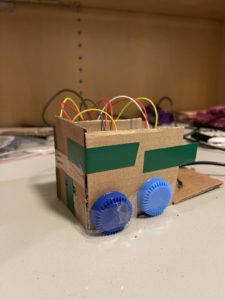So, I used the LEDs as the front light of the truck. Then, I had to use a switch, so I did that by using the back of the truck.

So, if I closed the back of the truck, one red LED would light up, if I opened it, the other would light up.

This is what the breadboard and Arduino looked like: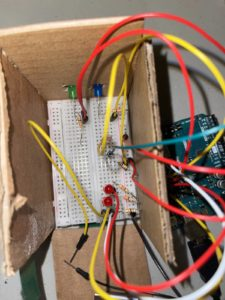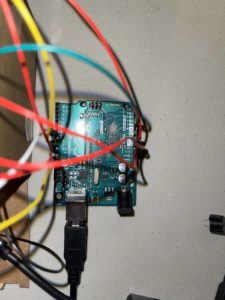This is how the LDR resistor looked like when it worked:

Overall, it was fun to use my creativity to learn how to use these tools.

The code for this is given below:

```const int ledPin = 10;
const int ledPin2 = 9;
const int knobPin = A1;

const int ledRed = 13;
const int switch1 = 7;
const int ledBlue = 12;
bool currentState = false;
bool switchState = LOW;

void setup() {
// put your setup code here, to run once:
Serial.begin(9600);
pinMode(ledPin, OUTPUT);
pinMode(ledPin2, OUTPUT);
pinMode(knobPin, INPUT);

pinMode(ledRed, OUTPUT);
pinMode(ledBlue, OUTPUT);
pinMode(switch1, INPUT);

}

void loop() {
// put your main code here, to run repeatedly:

if(ldrStatus <= 400){
digitalWrite(ledPin, HIGH);
digitalWrite(ledPin2, LOW);

}
else{
digitalWrite(ledPin, LOW);
digitalWrite(ledPin2, HIGH);

}

if (currentState != switchState ){
digitalWrite(ledBlue, LOW);
digitalWrite(ledRed, HIGH);
} else {
digitalWrite(ledBlue, HIGH);
digitalWrite(ledRed, LOW);
}
}```

## Week 8 – Sitting changes colour

Description

Create an unusual switch that doesn’t require the use of your hands. Use Arduino digital input and output for the interaction.

Process

So, I was just sitting on my chair trying to think about what to make. I got the idea, why don’t I just make something that involves sitting on the chair. So here it goes.

I used two LEDs ( red and blue). I used 3 resistors ( two 330 v and one 10k) to control the flow of electricity. I used basic conductors wires and jumper twins to finish the circuit. I connected the Red LED to Digital Input 13, Blue LED to Digital Input 12. I connected the switch to 7.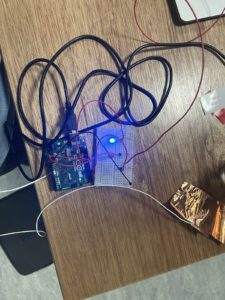I connected the copper wires to the copper tape to give it a bigger surface area to conduct electricity.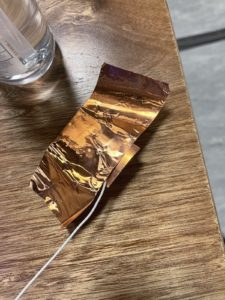This is the final video of me testing out the product:-

The code was pretty simple.

HERE IT GOES:-

```const int ledRed = 13;
const int switch1 = 7;
const int ledBlue = 12;
bool currentState = false;
bool switchState = LOW;

void setup() {
// put your setup code here, to run once:
pinMode(ledRed, OUTPUT);
pinMode(ledBlue, OUTPUT);
pinMode(switch1, INPUT);
}

void loop() {

///put your main code here, to run repeatedly:
if (currentState != switchState ){
digitalWrite(ledBlue, LOW);
digitalWrite(ledRed, HIGH);
} else {
digitalWrite(ledBlue, HIGH);
digitalWrite(ledRed, LOW);
}

}```

## COVID NINJA – Rhythm Kukreja’s Midterm Game

Inspiration:

As I mentioned in my proposal, my game was inspired by Fruit Ninja. Apart from slicing the fruits, you had to catch the covid objects, like pills, syringes, sanitizers, and masks. You also had to avoid catching the virus. There is also a powerup in the game, that looks like a clock, which slows the frameRate for about 5 seconds.

Process:

The game starts by showing a start screen, which gives the instructions for the game. Then, when you click any key on the keyboard, the game starts.

I used the keypressed function in order to switch from the start screen to the main game.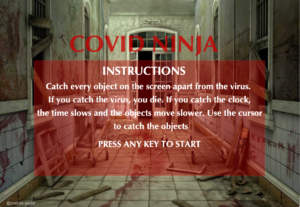After that, you can see a glove and objects falling from the top. The glove is made on mouse X and mouse Y. The objects use a random function on the width, to fall randomly from different sides and I have used gravity along with velocity to randomise the speed of the objects falling from the top.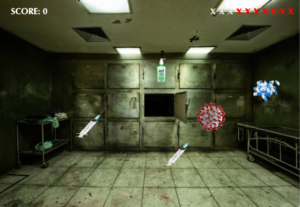Everytime, you catch the object, score increases by 1 and everytime you miss an object, the X on top gets red. If you miss 10 objects, the game ends. If you catch the virus game ends. I have used null to remove the objects.

Once the game ends, you see the following screen and I have again used keypressed function to restart the game, once the game ends.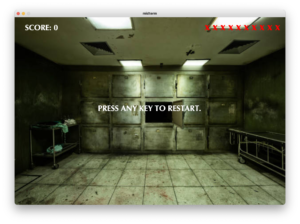This is what the game looks like:-

The code for the above game is below:-

```//setting up the variables, importing libraries, importing images and fonts.
import processing.sound.*;
SoundFile file;
SoundFile objects;
SoundFile bomb;
SoundFile gameover;
PImage back;
PImage knife;
PImage start;
PFont font;

int height = 720;
int width = 1040;
int frame = 0;

//initialising variables
Game game = new Game();
boolean gameOver = false;
boolean startScreen = true;

void setup(){
//setting up the screen and the background
size(1040, 720);
background(0);
font = createFont("Optima-Bold", 20);

//accessing sound path
file = new SoundFile(this, "main.wav");
objects = new SoundFile(this, "objects.mp3");
bomb = new SoundFile(this, "bomb.mp3");
gameover = new SoundFile(this, "gameover.mp3");

knife.resize(100,150);

start.resize(width,height);

//looping the background music
file.amp(0.05);
file.loop();
}

void draw(){
// setting font to optima bold
textFont(font);

//changing cursor settings
if(startScreen || gameOver){
cursor(CROSS);
} else {
cursor(knife);
}

// start screen for instructions
if(startScreen){
background(start);
strokeWeight(0);
fill(200,0,0,100);
rect((width/2)-400,(height/2)-150,800,400);

fill(200,0,0,200);
stroke(255);
textSize(80);
textAlign(CENTER);
text("COVID NINJA", (width/2)-20,(height/2)-180);

fill(255);
textSize(40);
textAlign(CENTER);
text("INSTRUCTIONS", (width/2)-20,(height/2)-100);

textSize(30);
textAlign(CENTER);
text("Catch every object on the screen apart from the virus. \n If you catch the virus, you die. If you catch the clock, \n the time slows and the objects move slower. Use the cursor \n to catch the objects", (width/2),(height/2)-50);
text("PRESS ANY KEY TO START",(width/2), (height/2)+150);
textAlign(BASELINE);

}

//condition to remove the start screen after keypressed
if(keyPressed){
startScreen = false;
}

//starting the game
if(!startScreen){
background(back);
//score change
fill(255); textSize(30); text("SCORE: ", 40, 50);
text(game.score, 150, 50);

//x's turing red after every miss
for(int i = 0; i < 10; i++){
if(i < game.missed){
fill(255,0,0);
}
else {
fill(220, 220, 220);
}
textSize(40);
text("x", width - 40 - (30*i), 50);
}

game.display();

//restarting the game after game is over
if(gameOver == true && keyPressed){
game = new Game();
frameRate(60);
gameOver = false;
}
}

}

//setting up class covid with variables
class Covid {
int x, y;
float vy, g;
boolean virus = false;
boolean clock = false;
PImage img;
int img_w, img_h;

Covid() {
x = (int) random(150, width-150);
y = 60;
g = random(0.1,0.3);
int selection = (int) random(1,60);
if(selection < 8){
selection = 6;
} else if(selection < 10) {
selection = 5;
} else {
selection = (selection%4) + 1;
}
if(selection == 6){
virus = true;
}
if(selection == 5){
clock = true;
}
img_w = 100; img_h = 100;
}

void gravity() {
vy = vy + g;
}

void update() {
gravity();
y += vy;
}

void display() {
update();
image(img, x, y, img_w, img_h);
}
}

class Game {
int difficulty;
int score;
int missed;
int numCovidItems;
boolean roundOver;
Covid[] covid;

//using the constructor
Game() {
difficulty = 1;
score = 0;
missed = 0;
roundOver = true;
}

//defining method
void updateCovid(){
//increasing the number of objects
difficulty++;
numCovidItems = difficulty;
covid = new Covid[numCovidItems];
for (int i=0; i<numCovidItems; i++){
covid[i] = new Covid();
}
}

//method display
void display(){
//condition for game over
if(missed >= 10){
// GAME OVER SCREEN
if (gameOver == false){
gameover.play();
}
gameOver = true;

textSize(30);
fill(255);
text("PRESS ANY KEY TO RESTART.", (width/2)-200, height/2);

} else {
if(frame+150 < frameCount){
frameRate(60);
}
if(roundOver == true){
updateCovid();
roundOver = false;
}

numCovidItems = difficulty;

//making the objects disappear
int killedobjs = 0;
for (int i=0; i<numCovidItems; i++){
if(covid[i] == null){
killedobjs++;
}
}
if(killedobjs == numCovidItems){
roundOver = true;
}

for (int i=0; i<numCovidItems; i++){
// checks if object is missed
if(covid[i] != null){
if(covid[i].y > height) {
if(!covid[i].virus) { missed++; }
covid[i] = null;
}
}
// checks if object is cut else displays
if(covid[i] != null){
if((covid[i].x <= (mouseX+50) && (mouseX-50) <= (covid[i].x + covid[i].img_w)) && (covid[i].y <= (mouseY+40) && (mouseY-50) <= (covid[i].y + covid[i].img_h))){
if(covid[i].virus) {
missed = 11;
bomb.play();
} else if (covid[i].clock) {
covid[i] = null;
frameRate(20);
frame = frameCount;
} else {
covid[i] = null;
score++;
objects.amp(0.1);
objects.play();
}
} else {
covid[i].display();
}
}
}
}
}
}```

## Midterm Progress (Covid Ninja) – Rhythm Kukreja

For our midterm project, we were tasked with making a game on Processing.

Inspiration

I was inspired by Fruit Ninja to make this kind of game. In fruit ninja, you slice fruits and avoid bombs.

Idea

My idea is to make a game that works similar to fruit ninja but instead of cutting fruits, we cut covid related things; like pills, vaccines, masks, etc and avoid cutting the virus. This game is called covid ninja.

Process

I started by assigning an X and Y variable that works for assigning the coordinates of the fruits. We want gravity in the covid objects, so we added randomize gravity between 0.1 and 0.5, which is further added with velocity to make it look like a free-falling object. Then, I will use a mouse pressed at the location of the object to slice the items. There will also be a slow time powerup which will come to a couple of times in the game for a few seconds to make the items go slower.

This is what the free-falling objects would look like for now:-

The code goes like this:-

```int height = 720;
int width = 1040;

String[] covidType = {"mask", "sanitizer", "virus", "vaccine", "pills"};
Game game = new Game();

void setup(){
size(1040, 720);
background(0);
}

void draw(){
background(0);
game.display();
}

class Covid {
int x, y;
float vy, g;
PImage img;
int img_w, img_h;

Covid() {
x = (int) random(150, width-150);
y = 0;
g = random(0.1,0.4);
img = loadImage(str((int) random(1,6)) + ".png");
img_w = 100; img_h = 100;
}

void gravity() {
vy = vy + g;
}

void update() {
gravity();
y += vy;
}

void display() {
update();
image(img, x, y, img_w, img_h);
}
}

class Game {
int difficulty;
int score;
int missed;
int numCovidItems;
boolean roundOver;
Covid[] covid;

Game() {
difficulty = 1;
score = 0;
missed = 0;
roundOver = true;
}

void updateCovid(){
numCovidItems = difficulty*3;
covid = new Covid[numCovidItems];
for (int i=0; i<numCovidItems; i++){
covid[i] = new Covid();
}
}

void display(){
if(roundOver == true){
updateCovid();
roundOver = false;
}
numCovidItems = difficulty*3;
for (int i=0; i<numCovidItems; i++){
covid[i].display();
}
}
}```

Description:

Create a generative typography/text output.

Process:

In the beginning, I just wanted to play with letters and characters. I thought I would use noise to make a pattern as I did in my previous assignment, but randomness in this assignment suited better. So I started by writing a simple text message in the center of the screen, then I split the message into characters by using an array. Then I played around to make the characters randomly move around the screen, so it looked like the letter was flying in the air. This is what it looked like.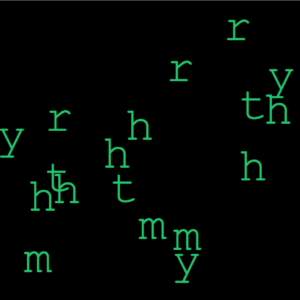Then, this looked boring, so I decided to add the key pressed function into it. So every time I would press the key pressed, my name would appear. The name would change its color and randomly disappear making it look nice and interactive. This is what it looked like.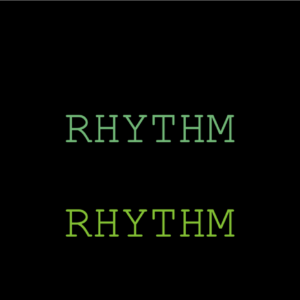Overall, I would say I wanted to try some other things as well, but I did not know the process for some things, so in the end, I ended up experimenting and made this.

The final video looks like this:

This is the entire code:

```// Intro to IM - Assignment #4
// Rhythm Kukreja (rk3781@nyu.edu)

//setting the variables below
String letter = "rhythmrhythmrhythm";
String letter2 = "RHYTHM";
PFont font;
float x,y;
float hr, vr;
float w = 40;
float h = 40;
float r = 100;
char[] charArray;

void setup(){
frameRate(10);//reducing the framerate for the random effect in the beginning
size(640, 640);

charArray = new char[letter.length()];//to separate the words by character
for(int i=0; i<letter.length(); i++){
charArray[i] = letter.charAt(i);
}

background(255);
hr = textWidth(letter) / 2;
vr = (textAscent() + textDescent()) / 2;
font = createFont("Courier New", 100, true);
textFont(font);
textAlign(CENTER);

x = height/2;
y = width/2;
}

void draw(){
fill(0);
rect(0, 0, width, height);
float xpos=0; float ypos=0;

if (keyPressed){// the key is pressed we see the text that changes color and disappers
for (int i = 0; i < letter2.length(); i++) {
int flag = int(random(0,100));
if(flag%2==1){
fill(random(0,200), random(180,200), random(50,180));
} else {
fill(0);
}
text(letter2, width/2, ((i*100)+100));
}

} else {    //if key is not pressed, then we see characters flying around randomly
fill(random(0,200), random(180,200), random(50,180));
for(int i=0; i<letter.length(); i++){
xpos = random(10,width-10); ypos = random(10,height-10);
text(charArray[i], xpos, ypos);
}
}
}```